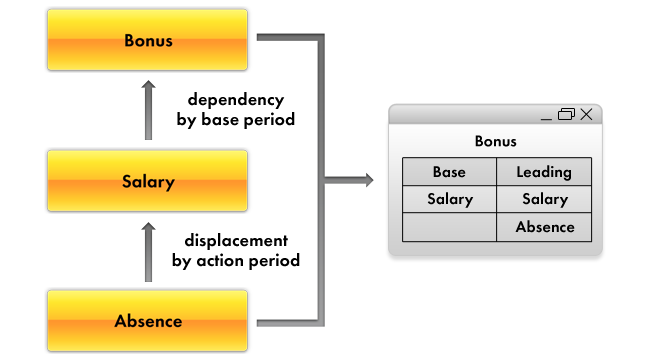# 1C:Enterprise 8.3. Practical Developer’s Guide. Lesson 17 (1:00). Charts of calculation types and calculation registers. Understanding charts of calculation types

## Understanding charts of calculation types

The Chart of calculation types configuration object is intended to define data storage structures for various calculation types. Based on a Chart of calculation types configuration object, the platform creates a database table that stores available calculation types and their interrelationships.

The distinctive characteristic of a chart of calculation types is that users can add new calculation types as they work. This feature makes the mechanism of periodic calculations more flexible and allows users to create their own calculation types in addition to those predefined by the developer.

The Chart of calculation types configuration object has the Uses Action Period property. If the property is set, the developer has the option to specify the calculation types that displace the current calculation type by action period.

Another important Chart of calculation types property is Dependence on Base. This property shows whether this chart of calculation types contains calculation types that are dependent by base period.

If the property is set, the developer can specify the chart of calculation types that stores the base calculation types, and also define the dependencies.

There are two types of base dependencies to choose from: dependency by action period and dependency by registration period. Both dependency types are discussed in detail in section Understanding calculation registers.

Another important feature of chart of calculation types is the option to create predefined calculation types and describe how they influence each other. In doing so, the developer can generally define three categories of calculation types that influence the predefined types:

• Base calculation types. Their results are used for recalculation of the current calculation type.
• Displacing calculation types. They displace the current calculation type by action period.
• Leading calculation types. Changes in their results force the recalculation of the current calculation type.

Common sense will tell you that all the leading calculation types should encompass all the base calculation types. Besides, leading calculation types can also contain some other calculation types that indirectly influence the current calculation type.

For example, there are three calculation types: Absence, Salary, and Bonus. Absence displaces Salary by action period, while Bonus depends on Salary by base period.

In this scenario, Salary should be the base calculation type for Bonus, and both Salary and Absence should be leading types for Bonus, since a change of the Absence calculation result forces a change of the Salary result, which in turn forces the change of the Bonus result (fig. 17.3).Fig. 17.3. Mutual influence of calculation types

Learn more! For details on the structure of 1C:Enterprise script objects intended for chart of calculation types operations, see section Quick developer reference. Charts of calculation types.